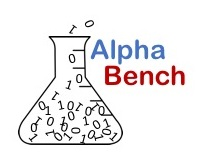## Linear Regression with SPSS

This tutorial demonstrates step-by step methods for running a simple linear regression in SPSS. Linear regression uses the values of one or more predictive variables, known as independent variable to predict the value of a dependent or response variable. This is sometimes referred to creating a linear model since the resulting equation of a line can be used to predict values of the dependent variable.

Linear regression relies on a number of assumptions to create a valid model.

## Regression Assumptions

1. variables being evaluated are on a continuous scale
2. a linear relationship exists
3. errors are not auto correlated
4. errors are approximately normally distributed
5. the dataset contains no outliers
6. variance of erros is roughly constant

This tutorial demonstrates both running the regression model and testing the regression assumptions to ensure model validity.